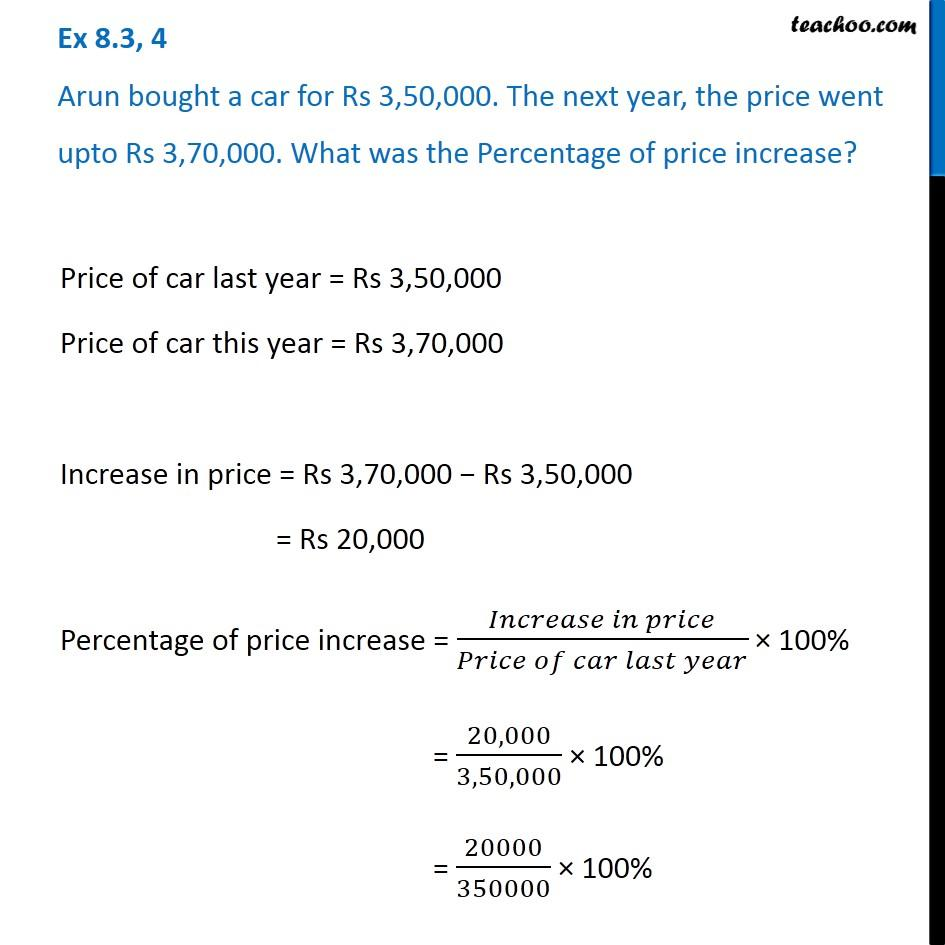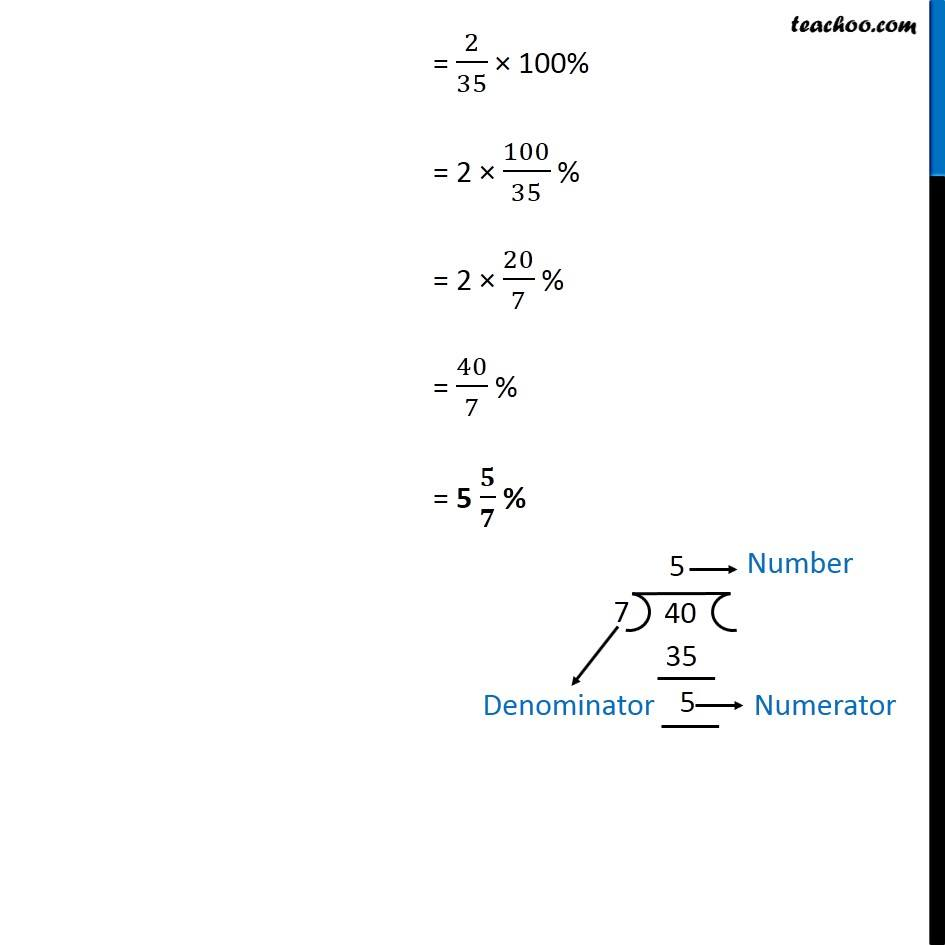Ex 8.3

Chapter 8 Class 7 Comparing Quantities
Serial order wiseGet live Maths 1-on-1 Classs - Class 6 to 12

### Transcript

Ex 8.3, 4 Arun bought a car for Rs 3,50,000. The next year, the price went upto Rs 3,70,000. What was the Percentage of price increase? Price of car last year = Rs 3,50,000 Price of car this year = Rs 3,70,000 Increase in price = Rs 3,70,000 − Rs 3,50,000 = Rs 20,000 Percentage of price increase = (𝐼𝑛𝑐𝑟𝑒𝑎𝑠𝑒 𝑖𝑛 𝑝𝑟𝑖𝑐𝑒)/(𝑃𝑟𝑖𝑐𝑒 𝑜𝑓 𝑐𝑎𝑟 𝑙𝑎𝑠𝑡 𝑦𝑒𝑎𝑟) × 100% = 20,000/3,50,000 × 100% = 20000/350000 × 100% = 2/35 × 100% = 2 × 100/35 % = 2 × 20/7 % = 40/7 % = 5 𝟓/𝟕 %